# Texas Go Math Grade 4 Lesson 14.1 Answer Key Angles and Fractional Parts

Refer to our Texas Go Math Grade 4 Answer Key Pdf to score good marks in the exams. Test yourself by practicing the problems from Texas Go Math Grade 4 Lesson 14.1 Answer Key Angles and Fractional Parts.

## Texas Go Math Grade 4 Lesson 14.1 Answer Key Angles and Fractional Parts

Essential Question

How can you relate angles and fractional ports of a circle?

Investigate

Materials

• fraction circles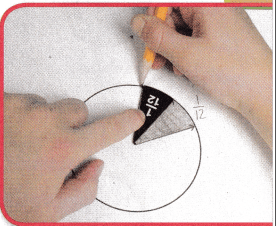A. Place a $$\frac{1}{12}$$ piece on the circle. Place the tip of the fraction piece on the center of the circle. Trace the fraction piece.
What figure is formed by the fraction piece? ______________

What parts of the fraction piece represent the rays of the angle? ______________

On what part of the circle is the vertex of the angle? ______________
The straight sides.
At the center of the circle.

Explanation:
Here, the parts of the fraction piece represent the rays of the angle are the straight sides.
At the center of the circle, the part of the circle is the vertex of the angle.

B. Shade the angle cut out by the $$\frac{1}{12}$$ piece. Label it $$\frac{1}{12}$$.
The shaded angle cut out by the $$\frac{1}{12}$$ piece is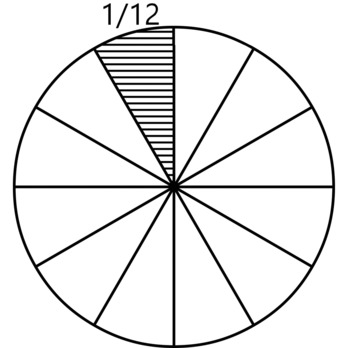C.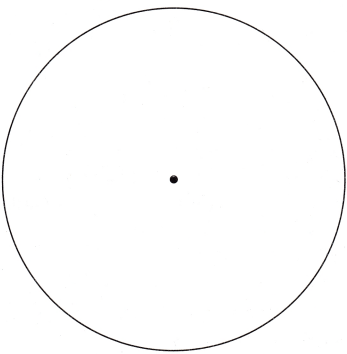Place the $$\frac{1}{12}$$ piece back on the shaded angle. Turn it counterclockwise. Counterclockwise is the direction opposite from the way the hands move on a clock.

Trace the fraction piece in its new position. How many twelfths have you traced in all? _______________ Label $$\frac{2}{12}$$.
2 traced twelfths.

Explanation:
By tracing the fraction piece in its new position we can see 2 traced twelfths.

D. Turn the fraction piece counter clockwise again and trace it. Label the total number of twelfths. Continue until you reach the shaded angle. What figure is formed by turning and tracing the fraction piece? _______________
How many times did you need to turn the $$\frac{2}{12}$$ piece to make a circle? _______________
How many angles came together in the center of the circle? _______________
12.

Explanation:
The number of times did we need to turn the $$\frac{2}{12}$$ piece to make a circle is 12.
The number of angles that came together in the center of the circle is 12.

Make Connections

You can relate fractions and angles to the hands of a clock.

Let the hands of the clock represent the rays of an angle that cuts out a fraction of the clock face. Each 5-minute mark represents a $$\frac{1}{12}$$ turn clockwise.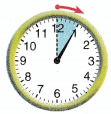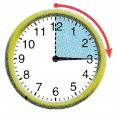15 minutes elapse.
The minute hand makes a
_____________ turn clockwise.
The minute hand makes a $$\frac{1}{4}$$ turn clockwise.

Explanation:
Here, minute hand makes a $$\frac{3}{12}$$ which is $$\frac{1}{4}$$ turn clockwise.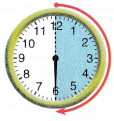30 minutes elapse.
The minute hand makes a
_____________ turn clockwise.
The minute hand makes a $$\frac{1}{2}$$ turn clockwise.

Explanation:
Here, minute hand makes a $$\frac{6}{12}$$ which is $$\frac{1}{2}$$ turn clockwise.45 minutes elapse.
The minute hand makes a
_____________ turn clockwise.
The minute hand makes a $$\frac{3}{4}$$ turn clockwise.

Explanation:
Here, minute hand makes a $$\frac{9}{12}$$ which is $$\frac{3}{4}$$ turn clockwise.60 minutes elapse.
The minute hand makes a
_____________ turn clockwise.
The minute hand makes a 1 turn clockwise.

Explanation:
Here, minute hand makes a $$\frac{12}{12}$$ which is 1 turn clockwise.

Share and Show

Look at the shaded part of the circle. Tell what fraction of the circle the angle cuts out.

Question 1.$$\frac{1}{2}$$.

Explanation:
The fraction of the circle the angle cuts out is $$\frac{1}{2}$$.

Question 2.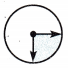$$\frac{1}{4}$$.

Explanation:
The fraction of the circle the angle cuts out is $$\frac{1}{4}$$.

Question 3.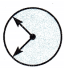$$\frac{3}{4}$$.

Explanation:
The fraction of the circle the angle cuts out is $$\frac{3}{4}$$.

Tell whether the angle on the circle shows a $$\frac{1}{4}, \frac{1}{2}, \frac{3}{4},$$ or 1 full turn clockwise or counterclockwise.

Question 4.$$\frac{1}{4}$$ turn counterclockwise.

Explanation:
Here, in the given image we can see that $$\frac{1}{4}$$ turns counterclockwise.

Question 5.$$\frac{1}{2}$$ turn clockwise.

Explanation:
Here, in the given image we can see that $$\frac{1}{2}$$ turns clockwise.

Question 6.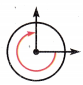$$\frac{3}{4}$$ turn clockwise.

Explanation:
Here, in the given image we can see that $$\frac{3}{4}$$ turns clockwise.

Problem Solving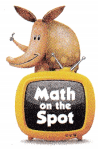H.O.T. Sense or Nonsense?Question 7.
Analyze Whose statement makes sense? Whose statement is nonsense? Explain your reasoning.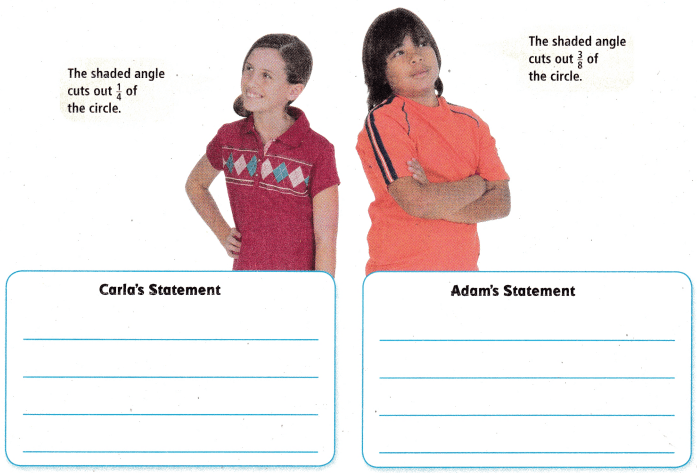Carla’s statement makes sense and Adam’s statement doesn’t make sense.

Explanation:
Here, Carla’s statement makes sense and Adam’s statement doesn’t make sense.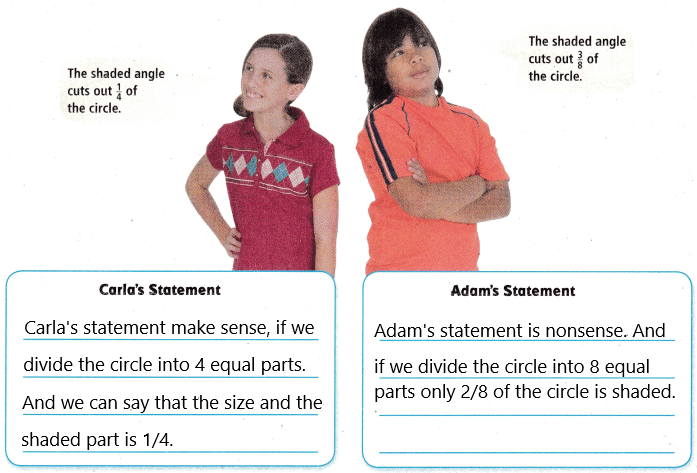Question 8.
H.O.T. Susan watched the game from 1 P.M. to 1:30 P.M.
Describe the turn the minute hand made.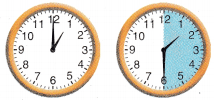$$\frac{1}{2}$$ turns clockwise.

Explanation:
Given that Susan watched the game from 1 P.M. to 1:30 P.M and the turn the minute hand made is $$\frac{1}{2}$$ turns clockwise.

Question 9.
Write Math Compare the angles in the two circles.
Does the position of the angle affect the size of the angle? Explain.No, the position of the angle affects the size of the angle.

Explanation:
No, the position of the angle affects the size of the angle. As both angles represent $$\frac{1}{2}$$ of the circle.

Question 10.
Multi-Step Carrie does her homework from 4 P.M. to 4:45 P.M.
Which describes the turn the minute hand makes?(A) $$\frac{1}{4}$$ turn clockwise
(B) $$\frac{3}{4}$$ turn clockwise
(C) $$\frac{1}{2}$$ turn counterclockwise
(D) $$\frac{1}{4}$$ turn counterclockwise
B.

Explanation:
Given that Carrie does her homework from 4 P.M. to 4:45 P.M, so the turn minute hand makes $$\frac{3}{4}$$ turn clockwise

Question 11.
Which angle shows $$\frac{1}{2}$$ turn counter cloclwise?
(A)(B)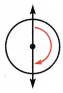(C)(D)B.

Explanation:
The angle that shows $$\frac{1}{2}$$ turn counter clockwise is B.

Question 12.
Look at the shaded part of the circle. What fraction of the circle does the angle cut out?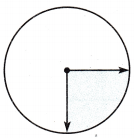(A) $$\frac{1}{4}$$
(B) $$\frac{1}{12}$$
(C) $$\frac{3}{4}$$
(D) $$\frac{1}{2}$$
A.

Explanation:
The fraction of the circle does the angle cut out $$\frac{1}{4}$$.

TEXAS Test Prep

Question 13.
Phillip watched a beach volleyball game from 1:45 RM. to 2:00 P.M.
What fraction of a turn did the minute hand make?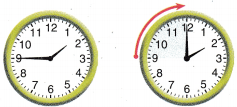(A) $$\frac{1}{4}$$ turn clockwise
(B) $$\frac{1}{2}$$ turn clockwise
(C) $$\frac{3}{4}$$ turn clockwise
(D) 1 full turn clockwise
C.

Explanation:
Given that Phillip watched a beach volleyball game from 1:45 PM. to 2:00 P.M. So the fraction of a turn did the minute hand make $$\frac{3}{4}$$ turn clockwise.

### Texas Go Math Grade 4 Lesson 15.1 Homework and Practice Answer Key

Look at the shaded part of the circle. Tell what fraction of the circle the angle cuts out.

Question 1.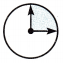$$\frac{1}{4}$$.

Explanation:
The fraction of the circle the angle cuts out is $$\frac{1}{4}$$.

Question 2.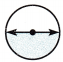$$\frac{1}{2}$$.

Explanation:
The fraction of the circle the angle cuts out is $$\frac{1}{2}$$.

Question 3.$$\frac{3}{4}$$.

Explanation:
The fraction of the circle the angle cuts out is $$\frac{3}{4}$$.

Tell whether the angle on the circle shows a $$\frac{1}{4}, \frac{1}{2}, \frac{3}{4}$$ or 1 full turn
clockwise or counterclockwise.

Question 4.$$\frac{3}{4}$$ turn clockwise.

Explanation:
Here, in the given image we can see that $$\frac{3}{4}$$ turns clockwise.

Question 5.$$\frac{1}{4}$$ turn clockwise.

Explanation:
Here, in the given image we can see that $$\frac{1}{4}$$ turns clockwise.

Question 6.1 turn clockwise.

Explanation:
Here, in the given image we can see that 1 turns clockwise.

Question 7.$$\frac{1}{2}$$ turn clockwise.

Explanation:
Here, in the given image we can see that $$\frac{1}{2}$$ turns clockwise.

Question 8.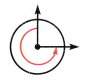Question 9.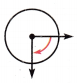$$\frac{1}{4}$$ turn clockwise.

Explanation:
Here, in the given image we can see that $$\frac{1}{4}$$ turns clockwise.

Problem Solving

Question 10.
Evan practiced the piano from 3 P.M. to 3:45 p.M. Describe the turn the minute hand made.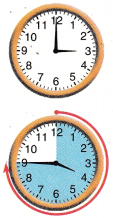$$\frac{3}{4}$$ turns clockwise.

Explanation:
Given that Evan practiced the piano from 3 P.M. to 3:45 P.M. So the turn the minute hand made is $$\frac{3}{4}$$ turns clockwise.

Question 11.
As soon as Evan finished practicing, he ate a snack. When he finished his snack, it was 4:00. Describe the turn the minute hand made.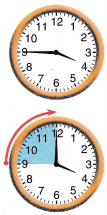$$\frac{1}{4}$$ turns clockwise.

Explanation:
As soon as Evan finished practicing, he ate a snack and it was 4:00, so the turn the minute hand made is $$\frac{1}{4}$$ turns clockwise.

Lesson Check

Question 12.
Which angle shows a $$\frac{3}{4}$$ turn counterclockwise?
(A)(B)(C)(D)C.

Explanation:
The image that shows an angle of $$\frac{3}{4}$$ turn counterclockwise is image C.

Question 13.
Look at the shaded part of the circle. What fraction of the circle does the angle cut out?(A) $$\frac{1}{2}$$
(B) $$\frac{3}{4}$$
(C) $$\frac{1}{3}$$
(D) $$\frac{1}{4}$$
D.

Explanation:
The fraction of the shaded part of the circle does the angle cut out is $$\frac{1}{4}$$.

Question 14.
Multi-Step Hannah takes a break from 10 A.M. to 10:15 A.M. Which describes the turn the minute hand makes?(A) $$\frac{1}{4}$$ turn countercIockvise
(B) $$\frac{1}{4}$$ turn clockwise
(C) $$\frac{3}{4}$$ turn counterclockwise
(D) $$\frac{3}{4}$$ turn clockwise
B.

Explanation:
Given that Hannah takes a break from 10 A.M. to 10:15 A.M. So the turn of the minute hand makes is $$\frac{1}{4}$$ turn clockwise.

Question 15.
Multi-Step Inez walks her dog each morning from 7:45 A.M. to 8:15 A.M. Which describes the turn the minute hand makes?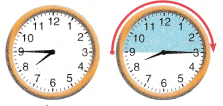(A) $$\frac{1}{2}$$ turn clockwise
(B) 1 full turn clockwise
(C) $$\frac{1}{2}$$ turn counterclockwise
(D) $$\frac{1}{4}$$ turn clockwise
A.

Explanation:
Given that Inez walks her dog each morning from 7:45 A.M. to 8:15 A.M, so the turn of the minute hand makes is $$\frac{1}{2}$$ turn clockwise.

Question 16.
Multi-Step Ben babysits his little sister for 15 minutes each day. Which fraction describes the turn of the minute hand on the clock face during the time Ben babysits?
(A) $$\frac{1}{2}$$
(B) $$\frac{3}{4}$$
(C) $$\frac{12}{12}$$
(D) $$\frac{1}{4}$$
D.

Expplanation:
Given that Ben babysits his little sister for 15 minutes each day, so the fraction of the turn of the minute hand on the clock face during the time Ben babysits is $$\frac{1}{4}$$.

Question 17.
Multi-Step Standard time begins in the fall. On a certain day each year, many people turn their clocks back one hour. Which fraction describes the turn of the minute hand on the clock face?
(A) $$\frac{12}{12}$$
(B) $$\frac{9}{12}$$
(C) $$\frac{3}{12}$$
(D) $$\frac{6}{12}$$
Given that on a certain day each year, many people turn their clocks back one hour, so the fraction that describes the turn of the minute hand on the clock face is $$\frac{12}{12}$$.